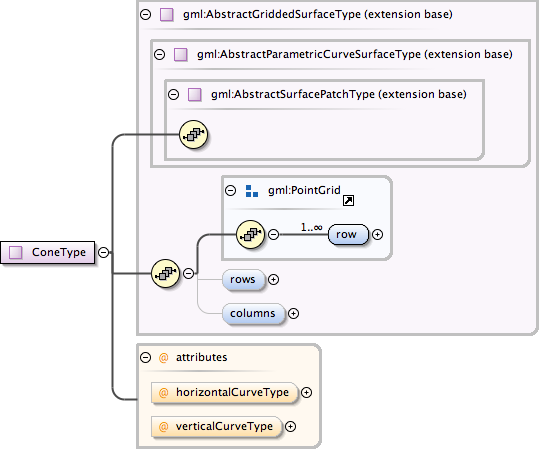### Showing:

 Annotations Attributes Diagrams Facets Properties Source Used by
Complex Type gml:ConeType
Namespace http://www.opengis.net/gml
Annotations
 ```A cone is a gridded surface given as a family of conic sections whose control points vary linearly. NOTE! A 5-point ellipse with all defining positions identical is a point. Thus, a truncated elliptical cone can be given as a 2x5 set of control points ((P1, P1, P1, P1, P1), (P2, P3, P4, P5, P6)). P1 is the apex of the cone. P2, P3,P4, P5 and P6 are any five distinct points around the base ellipse of the cone. If the horizontal curves are circles as opposed to ellipses, the a circular cone can be constructed using ((P1, P1, P1),(P2, P3, P4)). The apex most not coinside with the other plane.```
DiagramType extension of gml:AbstractGriddedSurfaceType
Type hierarchy
Used by
 Element gml:Cone
Model gml:row+ , gml:rows{0,1} , gml:columns{0,1}
Children gml:columns, gml:row, gml:rows
Attributes
QName Type Fixed Default Use Annotation
horizontalCurveType gml:CurveInterpolationType circularArc3Points optional
verticalCurveType gml:CurveInterpolationType linear optional
Source
 ``` A cone is a gridded surface given as a family of conic sections whose control points vary linearly. NOTE! A 5-point ellipse with all defining positions identical is a point. Thus, a truncated elliptical cone can be given as a 2x5 set of control points ((P1, P1, P1, P1, P1), (P2, P3, P4, P5, P6)). P1 is the apex of the cone. P2, P3,P4, P5 and P6 are any five distinct points around the base ellipse of the cone. If the horizontal curves are circles as opposed to ellipses, the a circular cone can be constructed using ((P1, P1, P1),(P2, P3, P4)). The apex most not coinside with the other plane. ```
Schema location http://schemas.opengis.net/gml/3.1.1/base/geometryPrimitives.xsd
Attribute gml:ConeType / @horizontalCurveType
Namespace No namespace
Type gml:CurveInterpolationType
Properties
 fixed: circularArc3Points
Facets
 enumeration linear enumeration geodesic enumeration circularArc3Points enumeration circularArc2PointWithBulge enumeration circularArcCenterPointWithRadius enumeration elliptical enumeration clothoid enumeration conic enumeration polynomialSpline enumeration cubicSpline enumeration rationalSpline
Used by
 Complex Type gml:ConeType
Source
 ``
Schema location http://schemas.opengis.net/gml/3.1.1/base/geometryPrimitives.xsd
Attribute gml:ConeType / @verticalCurveType
Namespace No namespace
Type gml:CurveInterpolationType
Properties
 fixed: linear
Facets
 enumeration linear enumeration geodesic enumeration circularArc3Points enumeration circularArc2PointWithBulge enumeration circularArcCenterPointWithRadius enumeration elliptical enumeration clothoid enumeration conic enumeration polynomialSpline enumeration cubicSpline enumeration rationalSpline
Used by
 Complex Type gml:ConeType
Source
 ``
Schema location http://schemas.opengis.net/gml/3.1.1/base/geometryPrimitives.xsd